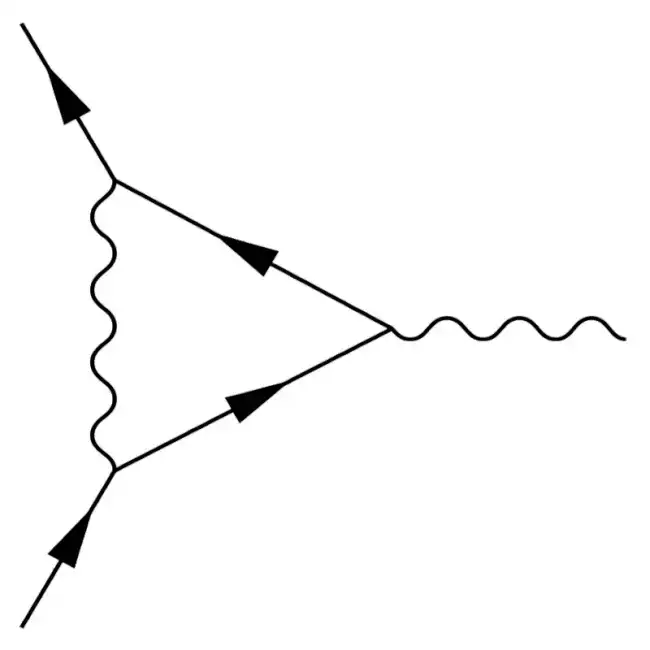## Quantum Field Theory

USOSThis is the mandatory Quantum Field Theory course of the Master in Theoretical Physics at the University of Wrocław. It is tailored towards master and PhD students who are familiar with

There are many good books on the subject, four that I like are

• [PeskSchr] Peskin, Schroeder: An introduction to quantum field theory
• [Ryder] Ryder: Quantum Field Theory
• Weinberg: The Quantum Theory of Fields, Volume 1 & 2
• Zee: Quantum Field Theory in a Nutshell.

We will follow mostly the first one in the lectures. There will be 2 hours of lectures and 2 hours of tutorial each week. Exercises will be posted here a week before the tutorial they are discussed in. Please keep in mind that active participation in the tutorials is important to pass the course. For more information, please refer to the syllabus (in Polish) or contact me directly.

Important: Students will be assigned to exercise problems by the system described below at the Thursday, 9:00 pm, before the tutorial. Please indicate your preferences by then.

Exam: As we decided together, the exam will be a written exam. It will take place on Tuesday, the 28th of June 2022 at 10:00 am in room 511. Note that we will start at this time! Please, be there a bit earlier to avoid problems. To prepare for the exam, you should have a look at the practise exam, which we will also discuss in the last tutorial. It may give you a better idea about possible questions and is intended to help you prepare.

Retake Exam: The retake exam will take place at Friday, the 9th of September 2022 10:00 am in room 447 (the same room where we had the lectures).

Additional material for the individual lectures, including the exercises which we discuss in the tutorials, is given below:

1. Reminder of spin 0 and $1/2$ fields

[PeskSchr] sections one and two

Please note that there are no exercise assignments for the first seminar. Instead, we continue with the lecture.

2. Reminder of spin 1 fields and abelian gauge symmetries

[Ryder] sections 3.3 and 4.4

3. Non-abelian gauge symmetries and Lie groups

[PeskSchr] section 15 except for 15.3 or alternatively [Ryder] sections 3.5 and 3.6

4. Path integral in quantum mechanics and for the scalar field

[PeskSchr] section 9.1

5. Generating functional, interactions and Feynman rules

[PeskSchr] section 9.2

6. Path integral for fermions, Grassmann numbers, chiral anomaly (in the exercise)

[PeskSchr] section 9.5

7. Path integral for spin-1 bosons, ghost fields

[PeskSchr] section 9.4

8. One loop effects in QED: field-strength renormalisation and self-energy

[PeskSchr] section 7.1

9. Dimensional regularisation and superficial degree of divergence

Peskin&Schroeder sections 7.5 and 10.1

10. One-loop renoramlised $\phi^4$ theory

[PeskSchr] section 10.2

11. Renormalisation group flow

[PresSchr] section 12.1

Note: Last time in the exercise, we discussed the renormalisation of Yukawa theory. In particular, we computed all counterterms at one loop. We did not finish this task, and I got the impression that at least some of you were struggling with this task. But in this exercise, we will need the counterterms to compute the β-functions. Therefore, we will not have a new problem set this week but instead finish the work on the counterterms together and discuss how we use them to compute the one-loop β-functions for Yukawa theory.

Please still prepare as well as possible by revisiting our problem sheet from last week.

12. The Callan-Symanzik equation and $\beta$-functions

[PresSchr] sections 12.2

As usual, we will assign students to the different subparts of this exercise. But it might be a good idea to try to solve it completely as a preparation for the exam. It contains most of the central concepts and techniques we learned in this course in a still tractable way.

13. Gravity, quantum gravity, one-loop $\beta$-functions of a two-dimensional $\sigma$-model and string theory
14. Spontaneous symmetry breaking and the Higgs mechanism

[PresSchr] sections 11.1 and 20.1

In the exercise, we discuss the practise exam. Also keep in mind that this is your last chance to hand in your bonus point problem about the one-loop β-functions of the non-linear σ-model (worth 6 additonal points).

15. BRST symmetry, physical Hilbert space

[PeskSchr] section 16.2-16.4

Based on course 4, last update on March 3rd 2023, 10:50:37 | Build 201 on May 26th 2023, 09:46:23 | Contact